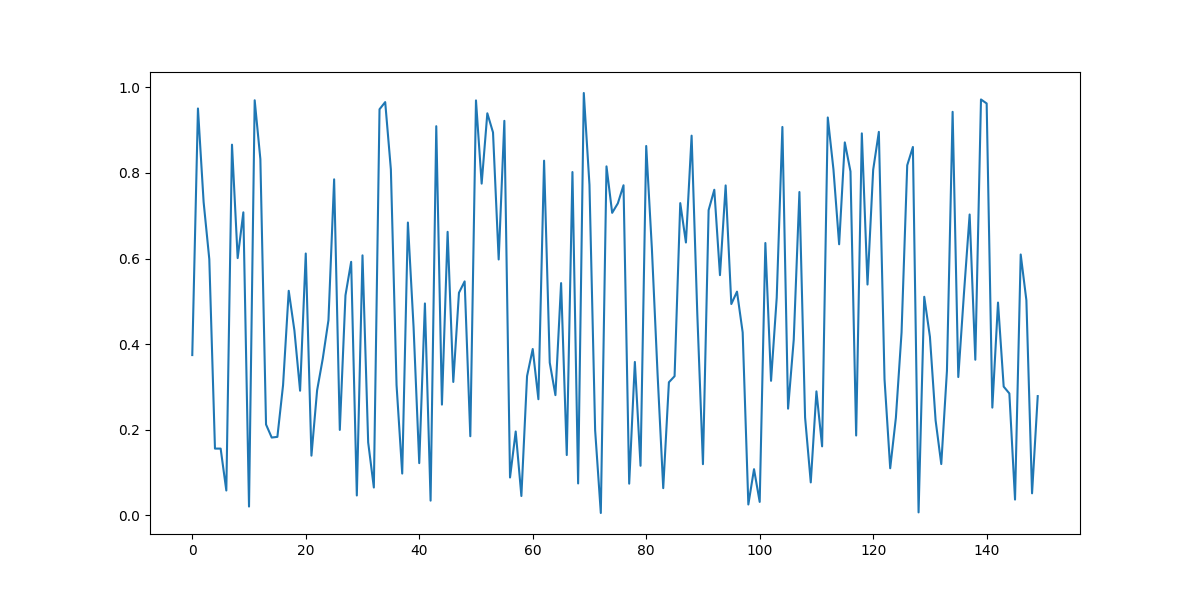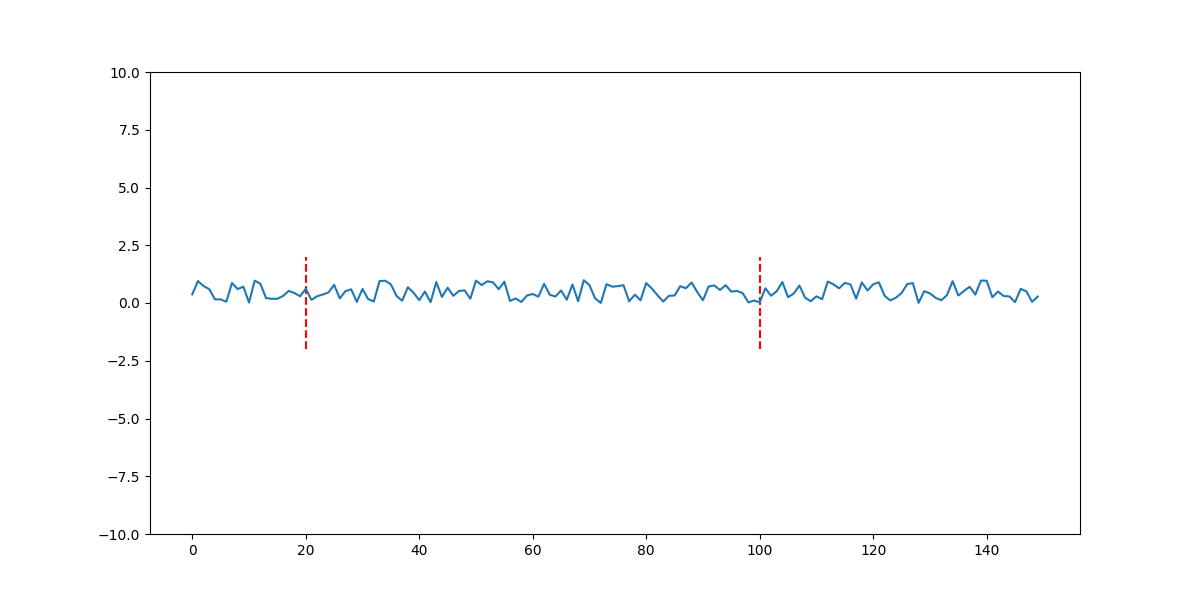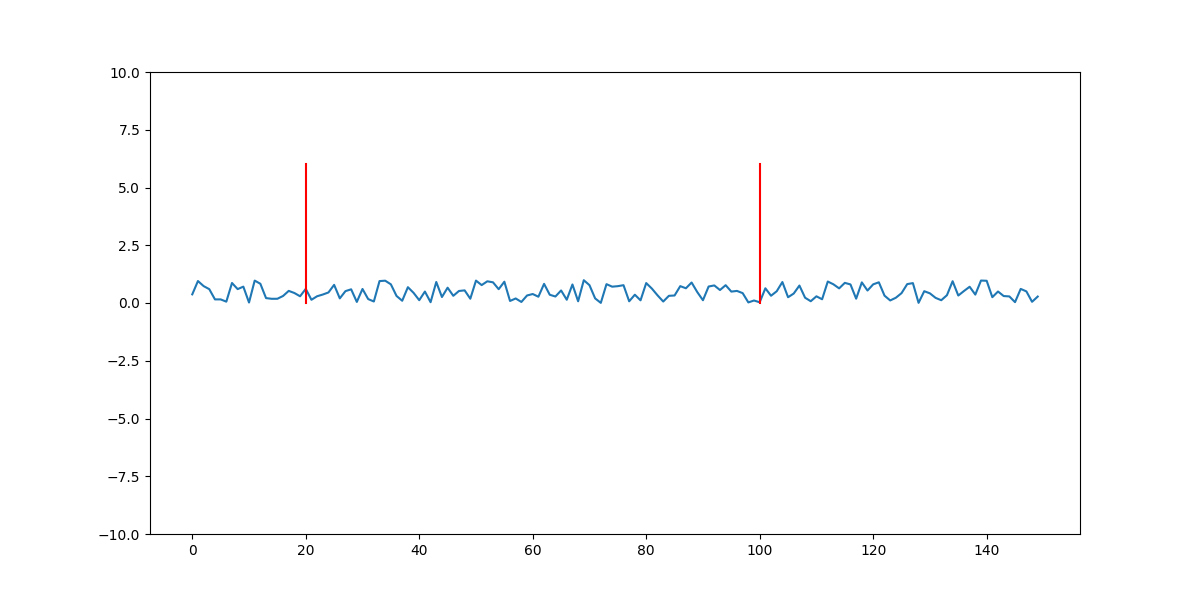Matplotlib: Draw Vertical Lines on Plot - Stack Abuse

# Matplotlib: Draw Vertical Lines on Plot### Introduction

Matplotlib is one of the most widely used data visualization libraries in Python. Much of Matplotlib's popularity comes from its customization options - you can tweak just about any element from its hierarchy of objects.

In this tutorial, we'll take a look at how to draw a vertical line on a Matplotlib plot, that allows us to mark and highlight certain regions of the plot, without zooming or changing the axis range.

### Creating a Plot

Let's first create a simple plot with some random data:

import matplotlib.pyplot as plt
import numpy as np

fig, ax = plt.subplots(figsize=(12, 6))

np.random.seed(42)
x = np.random.rand(150)
ax.plot(x)

plt.show()


Here, we've used Numpy to generate 150 random data points, in a range of [0, 1).Now, since we've set a seed, we can replicate this random image as many times as we'd like. For example, let's draw vertical lines on the 20 and 100 marks.

There are two ways we can draw lines, using the vlines() or axvline() functions of the PyPlot instance. Naturally, you can also call these methods on the Axes object.

### Draw Vertical Lines on Matplotlib Plot with PyPlot.vlines()

Let's start off with the vlines() function:

import matplotlib.pyplot as plt
import numpy as np

fig, ax = plt.subplots(figsize=(12, 6))

np.random.seed(42)
x = np.random.rand(150)
ax.plot(x)

ax.vlines([20, 100], 0, 1, linestyles='dashed', colors='red')

plt.show()


The vlines() function accepts a few arguments - a scalar, or 1D array of X-values that you'd like to draw lines on. We've supplied [20, 100], marking two points, though you can go from 0..n points here. Then, the ymin and ymax arguments - these are the height of the lines. We've set them to be from 0 to 1, since that's the distribution of the np.random.rand() call as well. Then, you can set styles, such as linestyles or colors, which accept the typical Matplotlib styling options.

Running this code will result in:We've got two vertical lines, which are dashed, in red color, at the 20 and 100 points on the X-axis.

This function allows us to set the ymin and ymax in concrete values, while axvline() lets us choose the height percentage-wise, or we simply let it plot from the bottom to the top by default.

This feature comes in handy when you'd like to make the lines shorter or longer, for example. Let's change the range of our Y-axis, to include the view from -10 to 10, instead of 0 and 1. Our random data will still be in the range from [0, 1) so we'll have a better look at it from a different perspective:

fig, ax = plt.subplots(figsize=(12, 6))

np.random.seed(42)
x = np.random.rand(150)
ax.plot(x)

ax.set_ylim(-10, 10)
ax.vlines([20, 100], -2, 2, linestyles='dashed', colors='red')Here, we've set the lines to be longer than the range of the random data itself, but still much smaller than the size of the Axes itself.

### Draw Vertical Lines on Matplotlib Plot with PyPlot.axvline()

Now, let's take a look at the axvline() function:

fig, ax = plt.subplots(figsize=(12, 6))

np.random.seed(42)
x = np.random.rand(150)
ax.plot(x)

ax.set_ylim(-10, 10)
ax.axvline(20, color='red')
ax.axvline(100, color='red')

plt.show()


It has a few limitations that the other function doesn't have, such as being able to only plot on a single point at a time. If we want to plot on multiple points, such as 20 and 100, we'll have to call the function twice.

## Better understand your data with visualizations

•  30-day no-questions refunds
•  Beginner to Advanced
•  Updated regularly (update June 2021)
•  New bonus resources and guides

It also doesn't really let us specify the linestyle like vlines() let us, though, it doesn't require the ymin and ymax arguments by default. If you omit them, like we have, they'll simply be from the top to the bottom of the Axes:However, you can change the height if you'd like - this time around though, you'll change the height in terms of percentages. These percentages take the top and bottom of the Axes into consideration so 0% will be at the very bottom, while 100% will be at the very top. Let's draw a line spanning from 50% to 80%:

fig, ax = plt.subplots(figsize=(12, 6))

np.random.seed(42)
x = np.random.rand(150)
ax.plot(x)

ax.set_ylim(-10, 10)
ax.axvline(20, 0.8, 0.5, color='red')
ax.axvline(100, 0.8, 0.5, color='red')


This produces:### Conclusion

In this tutorial, we've gone over how to draw vertical lines on a Matplotlib Plot.

If you're interested in Data Visualization and don't know where to start, make sure to check out our bundle of books on Data Visualization in Python:

Data Visualization in Python with Matplotlib and Pandas is a book designed to take absolute beginners to Pandas and Matplotlib, with basic Python knowledge, and allow them to build a strong foundation for advanced work with theses libraries - from simple plots to animated 3D plots with interactive buttons.

It serves as an in-depth, guide that'll teach you everything you need to know about Pandas and Matplotlib, including how to construct plot types that aren't built into the library itself.

Data Visualization in Python, a book for beginner to intermediate Python developers, guides you through simple data manipulation with Pandas, cover core plotting libraries like Matplotlib and Seaborn, and show you how to take advantage of declarative and experimental libraries like Altair. More specifically, over the span of 11 chapters this book covers 9 Python libraries: Pandas, Matplotlib, Seaborn, Bokeh, Altair, Plotly, GGPlot, GeoPandas, and VisPy.

It serves as a unique, practical guide to Data Visualization, in a plethora of tools you might use in your career.

Last Updated: April 12th, 2021

## Improve your dev skills!

Get tutorials, guides, and dev jobs in your inbox.

No spam ever. Unsubscribe at any time. Read our Privacy Policy.

# Prepping for an interview?

• Improve your skills by solving one coding problem every day
• Get the solutions the next morning via email
• Practice on actual problems asked by top companies, like:

# Better understand your data with visualizations

•  30-day no-questions refunds
•  Beginner to Advanced
•  Updated regularly (update June 2021)
•  New bonus resources and guides

© 2013-2021 Stack Abuse. All rights reserved.